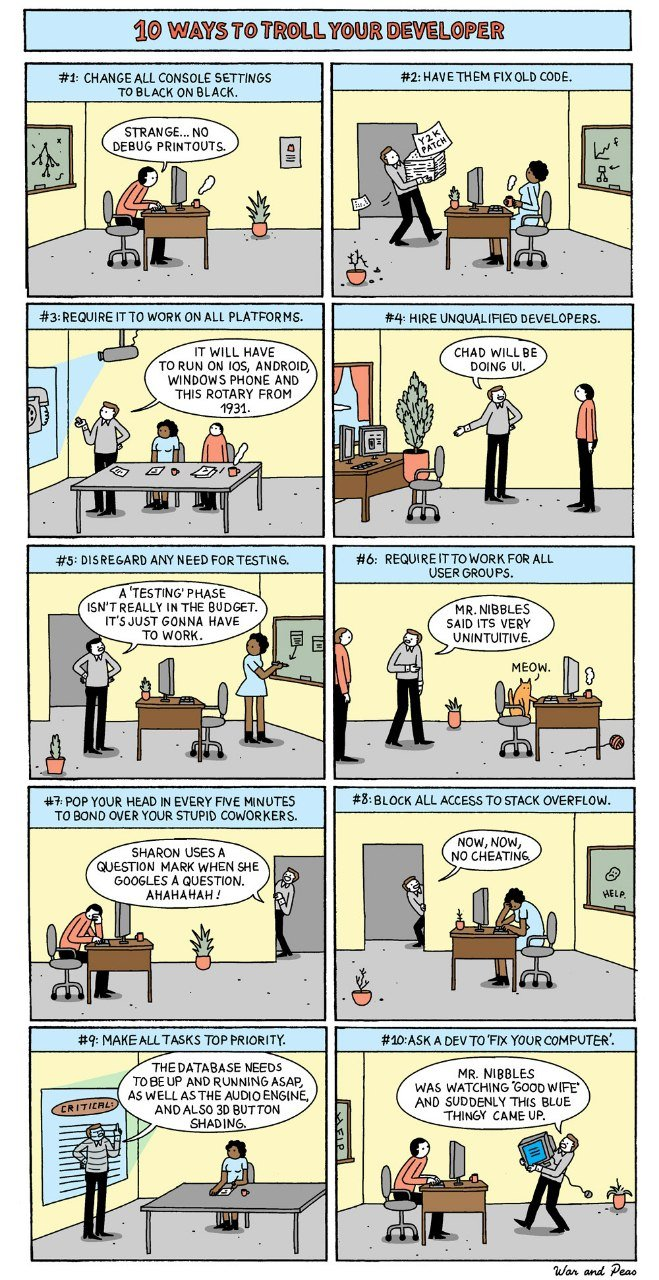## Kerkhoffs principle

Security information should rely on the secret and not the secrecy of the algorithm.

## ASCII ART

Generating ASCII art from images using Python3 and PIL (Python Image Library)

```
1pip install Pillow

```
```
1234567891011121314151617181920212223242526272829303132333435363738394041424344454647484950515253545556575859606162from PIL import Image
import os

ASCII_CHARS = &#91; '#', '?', '%', '.', 'S', '+', '.', '*', ':', ',', '@']

def scale_image(image, new_width=100):
"""Resizes an image preserving the aspect ratio.
"""
(original_width, original_height) = image.size
aspect_ratio = original_height/float(original_width)
new_height = int(aspect_ratio * new_width)

new_image = image.resize((new_width, new_height))
return new_image

def convert_to_grayscale(image):
return image.convert('L')

def map_pixels_to_ascii_chars(image, range_width=25):
"""Maps each pixel to an ascii char based on the range
in which it lies.

0-255 is divided into 11 ranges of 25 pixels each.
"""

pixels_in_image = list(image.getdata())
pixels_to_chars = &#91;ASCII_CHARS&#91;pixel_value//range_width] for pixel_value in
pixels_in_image]

return "".join(pixels_to_chars)

def convert_image_to_ascii(image, new_width=100):
image = scale_image(image)
image = convert_to_grayscale(image)

pixels_to_chars = map_pixels_to_ascii_chars(image)
len_pixels_to_chars = len(pixels_to_chars)

image_ascii = &#91;pixels_to_chars&#91;index: index + new_width] for index in
range(0, len_pixels_to_chars, new_width)]

return "\n".join(image_ascii)

def handle_image_conversion(image_filepath):
image = None
try:
image = Image.open(image_filepath)
except Exception as e:
print("Unable to open image file {image_filepath}.".format(image_filepath=image_filepath))
print(e)
return

image_ascii = convert_image_to_ascii(image)
f = open(os.path.splitext(image_filepath)&#91;0]+'.txt','w')
f.write(image_ascii)
f.close()

if __name__=='__main__':
import sys

image_file_path = 'your_path/to_your_image/goes_here.png'
handle_image_conversion(image_file_path)

```

## St. Ignucious in Cyprus

The photo is from the Richard Stallman (RMS) visit to Cyprus. We are both staring and not starting at the ancient settlement of Choirokoitia.
You can listen to his speech here

Copyright vs Community in the Age of Computer Networks
https://archive.org/details/rmsCyprus

## 10 ways to troll your developer.